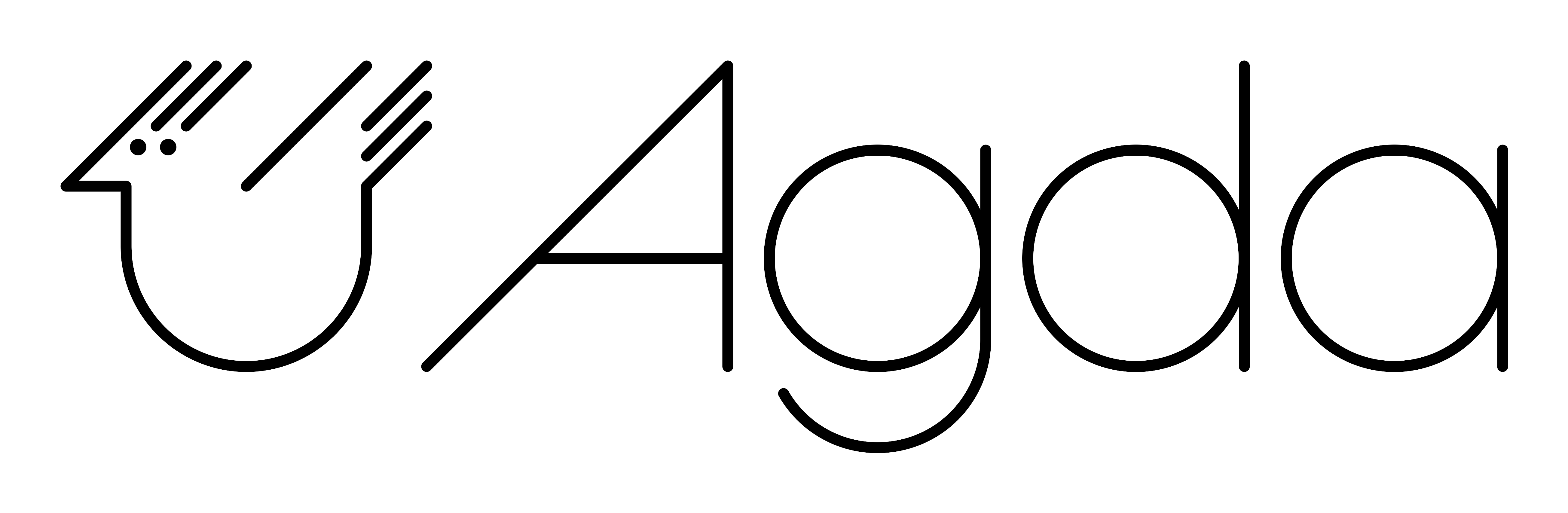# What is Agda?¶Agda is a dependently typed programming language. It is an extension of Martin-Löf’s type theory and is the latest in the tradition of languages developed in the programming logic group at Chalmers. Other languages in this tradition are Alf, Alfa, Agda 1, Cayenne. Some other loosely related languages are Coq, Epigram, and Idris.

Because of strong typing and dependent types, Agda can be used as a proof assistant, allowing to prove mathematical theorems (in a constructive setting) and to run such proofs as algorithms.

## Dependent types¶

### Typing for programmers¶

Type theory is concerned both with programming and logic. We see the type system as a way to express syntactic correctness. A type correct program has a meaning. Lisp is a totally untyped programming language, and so are its derivatives like Scheme. In such languages, if `f` is a function, one can apply it to anything, including itself. This makes it easy to write programs (almost all programs are well formed), but it also makes it easy to write erroneous programs. Programs will raise exceptions or loop forever. And it is very difficult to analyze where the problems are.

Haskell or ML and its derivatives like Standard ML and Caml are typed languages, where functions come with a type expressing what type of arguments the program expects and what the result type is.

Between these two families of languages come languages, which may or may not have a typing discipline. Most imperative languages do not come with a rich type system. For example, C is typed, but very loosely (almost everything is an integer or a variant thereof). Moreover, the typing system does not allow the definition of trees or graphs without using pointers.

All these languages are examples of partial languages, i.e., the result of computing the value of an expression `e` of type `T` is one of the following:

• the program terminates with a value in the type `T`
• the program `e` does not terminate
• the program raises an exception (which has been caused by an incomplete definition – for instance, a function is only defined for positive integers but is applied to a negative integer.

Agda and other languages based on type theory are total languages in the sense that a program `e` of type `T` will always terminate with a value in `T`. No runtime error can occur, and no nonterminating programs can be written (unless explicitly requested by the programmer).

### Dependent types¶

Dependent types are introduced by having families of types indexed by objects in another type. For instance, we can define the type `Vec n` of vectors of length `n`. This is a family of types indexed by objects in `Nat` (a type parameterized by natural numbers).

Having dependent types, we must generalize the type of functions and the type of pairs.

The dependent function space `(a : A) -> (B a)` is the type of the functions taking an argument `a` in a type `A` and a result in ```B a```. Here, `A` is a type, and `B` is a family of types indexed by elements in `A`.

For example, we could define the type of `n x m` matrices as a type indexed by two natural numbers. Call this type `Mat n m`. The function `identity`, which takes a natural number `n` as an argument and produces the `n x n` identity matrix, is then a function of type `identity : (n : Nat) -> (Mat n n)`.

Remark: We could, of course, just specify the `identity` function with the type `Nat -> Nat -> Mat`, where `Mat` is the type of matrices, but this is not as precise as the dependent version.

The advantage of using dependent types is that it makes it possible to express properties of programs in the typing system. We saw above that it is possible to express the type of square matrices of length `n`. It is also possible to define the type of operations on matrices so that the lengths are correct. For instance, the type of matrix multiplication is

```∀ {i j k} → (Mat i j) -> (Mat j k) -> (Mat i k)
```

and the type system can check that a program for matrix multiplication really takes arguments of the correct size. It can also check that matrix multiplication is only applied to matrices, where the number of columns of the first argument is the same as the number of rows in the second argument.

### Dependent types and logic¶

Thanks to the Curry-Howard correspondence, one can express a logical specification using dependent types. For example, using only typing it is possible to define:

• equality on natural numbers
• properties of arithmetical operations
• the type `(n : Nat) -> (PrimRoot n)` consisting of functions computing primitive root in modular arithmetic.

Of course, a program of the above type will be more difficult to write than the corresponding program of type `Nat -> Nat`, which produces a natural number which is a primitive root. However, the difficulty can be compensated by the fact that the program is guaranteed to work: it cannot produce something which is not a primitive root.

On a more mathematical level, we can express formulas and prove them using an algorithm. For example, a function of type ```(n : Nat) -> (PrimRoot n)``` is also a proof that every natural number has a primitive root.